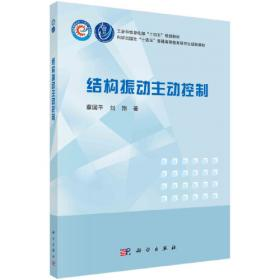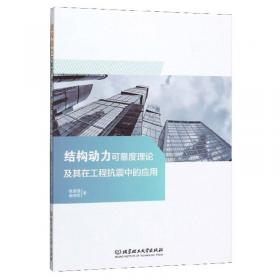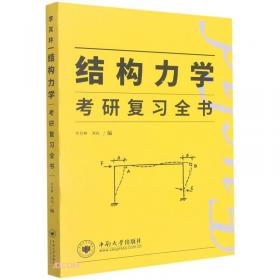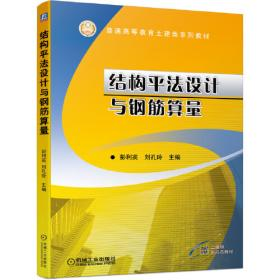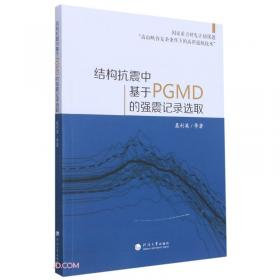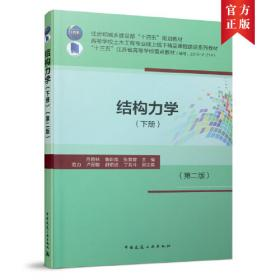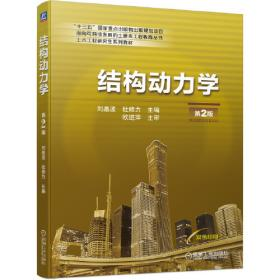# 结构动力学基础（英文版）2021-05

ISBN: 9787548737810

• 内容提要：《结构动力学基础》介绍了结构振动的基本概念与基本原理。本书共分为七章，具体内容为：结构动力学概述；系统运动方程的建立；单自由度系统动力响应分析；多自由度系统动力响应分析；连续系统（直梁）动力响应分析；固有频率与振型的近似分析方法；逐步积分法。每一章均包含了一些拓展型的实例与思考题，便于读者加深对基本理论的理解。
主要特点：
本书包含曾庆元院士提出的两项原创性成果，即弹性系统动力学总势能不变值原理与形成系统矩阵的“对号入座”法则。
在上述原理与方法的基础上，本书作者提出了基于Matlab语言形成系统矩阵的程序实现方法，方便读者编写动力有限元计算程序。
本书内容简练，涵盖结构动力学的主要知识点，便于读者掌握结构动力学的基本概念与分析方法。
本书所提供的结构动力学基本知识与一些原创性成果，可为有关的学生、研究人员以及工程师提供参考。 Contents

Chapter 1  Overview of Structural Dynamics   1

1.1  Objective of structural dynamic analysis   1

1.2  Characteristics of structural dynamics   2

1.3  Classification of vibrations   3

1.4  Vibration problems in engineering   5

1.5  Procedures of dynamic response analysis of structures  6

Problems  9

References  9

Chapter 2  Formulation of Equations of Motion of Systems  10

2.1  System constraints  10

2.2  Representation of system configuration  13

2.3  Real displacements， possible displacements and virtual displacements  16

2.4  Generalized force  19

2.5  Conservative force and potential energy  23

2.6  Direct equilibrium method  25

2.7  Principle of virtual displacements  26

2.8  Lagranges equation  29

2.9  Hamiltons principle  33

2.10  Principle of total potential energy with a stationary value in elastic system dynamics

37

2.11  The ‘set-in-right-position’ rule for assembling system matrices and method of

computer implementation in Matlab  44

Problems  57

References  59

Chapter 3  Analysis of Dynamic Response of SDOF Systems  60

3.1  Analysis of free vibrations  60

3.2  Response of SDOF systems to harmonic loads  71

3.3  Vibration caused by base motion and vibration isolation81

3.4  Introduction to damping theory87

3.5  Evaluation of viscousdamping ratio  91

3.6  Response of SDOF systems to periodic loads  97

3.7  Response of SDOF systems to impulsive loads  99

3.8  Time domain analysis of dynamic response to arbitrary dynamic loads 111

3.9  Frequency domain analysis of dynamic response to arbitrary dynamic loads 113

Problems  118

References  120

Chapter 4  Analysis of Dynamic Response of MDOF Systems： Mode Superposition Method  121

4.1  Analysis of dynamic properties of MDOF systems  121

4.2  Coupling characteristics and uncoupling procedure of equations of MDOF systems

127

4.3  Analysis of free vibration response of undamped systems  132

4.4  Response of undamped systems to arbitrary dynamic loads  135

4.5  Response of damped systems to arbitrary dynamic loads  137

Problems  143

References  144

Chapter 5  Analysis of Dynamic Response of Continuous Systems： Straight beam  145

5.1  Differential equations of motion of undamped straight beam  146

5.2  Modal expansion of displacement and orthogonality of mode shapes of straight beam

147

5.3  Free vibration analysis of undamped straight beam  151

5.4  Forced vibration analysis of undamped straight beam   155

5.5  Forced vibration analysis of damped straight beam  158

Problems  161

References  162

Chapter 6  Approximate Evaluation of Natural Frequencies and Mode Shapes  163

6.1  Rayleigh energy method  163

6.2  Rayleigh-Ritz method 168

6.3  Matrix iteration method 172

6.4  Subspace iteration method 177

6.5  Reduction of degrees of freedom in dynamic analysis 183

Problems 187

References 188

Chapter 7  Step-by-step Integration Method  189

7.1  Basic idea of step-by-step integration method  189

7.2  Linear acceleration method191

7.3  Wilson-θ method 195

7.4  Newmark  method  197

7.5  Stability and accuracy of stepbystep integration method  199

Problems 206

References 206
• ##### 内容简介:
内容提要：《结构动力学基础》介绍了结构振动的基本概念与基本原理。本书共分为七章，具体内容为：结构动力学概述；系统运动方程的建立；单自由度系统动力响应分析；多自由度系统动力响应分析；连续系统（直梁）动力响应分析；固有频率与振型的近似分析方法；逐步积分法。每一章均包含了一些拓展型的实例与思考题，便于读者加深对基本理论的理解。
主要特点：
本书包含曾庆元院士提出的两项原创性成果，即弹性系统动力学总势能不变值原理与形成系统矩阵的“对号入座”法则。
在上述原理与方法的基础上，本书作者提出了基于Matlab语言形成系统矩阵的程序实现方法，方便读者编写动力有限元计算程序。
本书内容简练，涵盖结构动力学的主要知识点，便于读者掌握结构动力学的基本概念与分析方法。
本书所提供的结构动力学基本知识与一些原创性成果，可为有关的学生、研究人员以及工程师提供参考。
• ##### 目录:
Contents

Chapter 1  Overview of Structural Dynamics   1

1.1  Objective of structural dynamic analysis   1

1.2  Characteristics of structural dynamics   2

1.3  Classification of vibrations   3

1.4  Vibration problems in engineering   5

1.5  Procedures of dynamic response analysis of structures  6

Problems  9

References  9

Chapter 2  Formulation of Equations of Motion of Systems  10

2.1  System constraints  10

2.2  Representation of system configuration  13

2.3  Real displacements， possible displacements and virtual displacements  16

2.4  Generalized force  19

2.5  Conservative force and potential energy  23

2.6  Direct equilibrium method  25

2.7  Principle of virtual displacements  26

2.8  Lagranges equation  29

2.9  Hamiltons principle  33

2.10  Principle of total potential energy with a stationary value in elastic system dynamics

37

2.11  The ‘set-in-right-position’ rule for assembling system matrices and method of

computer implementation in Matlab  44

Problems  57

References  59

Chapter 3  Analysis of Dynamic Response of SDOF Systems  60

3.1  Analysis of free vibrations  60

3.2  Response of SDOF systems to harmonic loads  71

3.3  Vibration caused by base motion and vibration isolation81

3.4  Introduction to damping theory87

3.5  Evaluation of viscousdamping ratio  91

3.6  Response of SDOF systems to periodic loads  97

3.7  Response of SDOF systems to impulsive loads  99

3.8  Time domain analysis of dynamic response to arbitrary dynamic loads 111

3.9  Frequency domain analysis of dynamic response to arbitrary dynamic loads 113

Problems  118

References  120

Chapter 4  Analysis of Dynamic Response of MDOF Systems： Mode Superposition Method  121

4.1  Analysis of dynamic properties of MDOF systems  121

4.2  Coupling characteristics and uncoupling procedure of equations of MDOF systems

127

4.3  Analysis of free vibration response of undamped systems  132

4.4  Response of undamped systems to arbitrary dynamic loads  135

4.5  Response of damped systems to arbitrary dynamic loads  137

Problems  143

References  144

Chapter 5  Analysis of Dynamic Response of Continuous Systems： Straight beam  145

5.1  Differential equations of motion of undamped straight beam  146

5.2  Modal expansion of displacement and orthogonality of mode shapes of straight beam

147

5.3  Free vibration analysis of undamped straight beam  151

5.4  Forced vibration analysis of undamped straight beam   155

5.5  Forced vibration analysis of damped straight beam  158

Problems  161

References  162

Chapter 6  Approximate Evaluation of Natural Frequencies and Mode Shapes  163

6.1  Rayleigh energy method  163

6.2  Rayleigh-Ritz method 168

6.3  Matrix iteration method 172

6.4  Subspace iteration method 177

6.5  Reduction of degrees of freedom in dynamic analysis 183

Problems 187

References 188

Chapter 7  Step-by-step Integration Method  189

7.1  Basic idea of step-by-step integration method  189

7.2  Linear acceleration method191

7.3  Wilson-θ method 195

7.4  Newmark  method  197

7.5  Stability and accuracy of stepbystep integration method  199

Problems 206

References 206